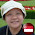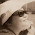## Saturday, 2 March 2013

### 6 AM Quiz: Let's Test FIDO out!

In this round of 6 am quiz, you will attempt to uncover the mystery behind Fido's Mind-Reading ability.

There are several ways to explain Fido's trick, using mathematical reasoning.
Make an attempt to use ALGEBRA to explain the "trick"?

1.For the fido puzzle, the sum of digits after you subtract the “jumbled up” numbers from the original number or the original number from the “jumbled up” numbers will always be a multiple of 9. So since it is a multiple of 9, the system/Fido can predict which multiple you are referring to rounding up the sum of the digits to the nearest multiple of 9 and subtract the numbers (digits) given by you accordingly.Then the answer is the one that you circled.

Using numbers:
So example, original number is 1234 and “jumbled up” number is 4321, you have to subtract 1234 from 4321, and answer is 3087. The sum of 3, 8 and 7 is 18, which a multiple of 9. If you circled 3, you will give the system/Fido 8 and 7. Since 8 and 7 added together is 15, the nearest multiple of 9 (rounded up) is 18, the system/Fido will subtract 15 from 18. 15-18=3. So the system/Fido will know that you chose 3.

Using algebra:
Assuming qwer are digits of the number after you had subtract the “jumbled up” numbers from the original number or the original number from the “jumbled up” numbers. q+w+e+r=multiple of 9. If q is circled, you have to give the system/Fido w, e and r to the system/Fido and the system/Fido will add w, e and r together and round up the number to the nearest multiple of 9 (x) and the subtract (w+e+r) from x to get q.

2.For example, take each of the four numbers from the four digit number to be a, b, c and d.

Therefore,

The four digit number is 1000a + 100b + 10c + d

Here is a random example of the jumbled up numbers [ from “a b c d” to “c a d b” ]

⇒ 1000c + 100a + 10d + b

so if “ a b c d” is the greater number, the difference of 1000a + 100b + 10c + d and 1000c + 100a + 10d + b would be …

[ 1000a – 100a ] + [ 100b – b ] + [ 10c – 1000c ] + [ d-10d ]
= 900a + 99b + [ -990c ] + [ -9d ]

all the coefficients of the variables are multiples of 9 , thus knowing that after adding up each number in the difference, you would always get the multiple of 9.

After typing in the 3 jumbled up numbers is the end, the computer would just have to add each number and find the total. After finding the total, the computer would just have to subtract it from the nearest multiple of 9 that is higher than the number itself and the difference would be the number that you have circled : ]

TADAH !!!! the magic of maths :P

3.I wish there's a like button in the blogs' comments as a response to the rssponses written above :)

4.To stretch the thinking behind a bit more...
Just wondering... Do you think Fido can handle numbers that go beyond 4 digits?

5.yes, he can. After repeating the same method that i have stated above [ it would always be (multiple of 9) x e ( pretend that e is the 5th number ) ]he would just have to add up each number typed in to the box and again subtract it from the nearest multiple of 9 that is higher than the total itself

6.Claire nice one.
Here is mine:
(1000a+100b+10c+d)-(1000b+100c+10a+d)= -900b+(-90c)+990a

-900b+(-90c)+990a is a multiple of 9 and it will always be so. One can go ahead and try it out with any combination of a,b,c and d. I will continue with my combination.

With the 3 jumbled digits that the computer gets, it will add them up and subtract them from the lowest multiple of 9 that is larger than the number that the computer gets. Thus, the computer can find the missing digit. This can be done for as many digits as one desires. I have performed this trick with six or seven digits with my mom before.

7.lets take the number abcd.
a is 1000a
b is 100b
c is 10c
d is 1d
if we jumble up the numbers, like bcda,
then (1000a + 100b + 10c + 1d) - (1000b + 100c + 10d + 1a) = 999a + 900b + 90c + 9d
All the numbers are multiples of 9.

8.When you come up with the 2 digits and you minus them, you will end up with a number.If you add up all the digits in the number, you will get 18.So when Fido asks you to write the numbers out except for the number you circled, Fido will take 18 minus the digits you have given and lo and behold, the number you have circled will be displayed in Fido's hand.

9.Oh i am sorry dont take my first reasoning i have discovered that it is wrong

1.Sandeep, why not reply to your original posting and tell us what's wrong with the 1st reasoning (what make you think it's wrong?)